Theory and Modern Applications

# On a k-Order System of Lyness-Type Difference Equations

## Abstract

We consider the following system of Lyness-type difference equations: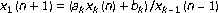,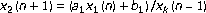,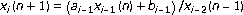,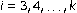, where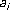,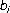,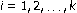, are positive constants,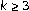is an integer, and the initial values are positive real numbers. We study the existence of invariants, the boundedness, the persistence, and the periodicity of the positive solutions of this system.

## References

1. Clark D, Kulenović MRS: A coupled system of rational difference equations. Computers & Mathematics with Applications 2002,43(6–7):849–867. 10.1016/S0898-1221(01)00326-1

2. Clark D, Kulenović MRS, Selgrade JF: Global asymptotic behavior of a two-dimensional difference equation modelling competition. Nonlinear Analysis: Theory, Methods, & Applications 2003,52(7):1765–1776. 10.1016/S0362-546X(02)00294-8

3. Kulenović MRS, Merino O: Discrete Dynamical Systems and Difference Equations with Mathematica. Chapman & Hall/CRC, Boca Raton, Fla, USA; 2002:xvi+344.

4. Kulenović MRS, Nurkanović Z: Global behavior of a three-dimensional linear fractional system of difference equations. Journal of Mathematical Analysis and Applications 2005,310(2):673–689.

5. Kulenović MRS, Nurkanović M: Asymptotic behavior of a competitive system of linear fractional difference equations. Advances in Difference Equations 2006, 2006: 13 pages.

6. Papaschinopoulos G, Schinas CJ: Invariants for systems of two nonlinear difference equations. Differential Equations and Dynamical Systems 1999,7(2):181–196.

7. Papaschinopoulos G, Schinas CJ: On the behavior of the solutions of a system of two nonlinear difference equations. Communications on Applied Nonlinear Analysis 1998,5(2):47–59.

8. Schinas CJ: Invariants for difference equations and systems of difference equations of rational form. Journal of Mathematical Analysis and Applications 1997,216(1):164–179. 10.1006/jmaa.1997.5667

9. Sun T, Xi H, Hong L: On the system of rational difference equations . Advances in Difference Equations 2006, 2006: 7 pages.

10. Sun T, Xi H: On the system of rational difference equations . Advances in Difference Equations 2006, 2006: 8 pages.

11. Kocić VL, Ladas G: Global Behavior of Nonlinear Difference Equations of Higher Order with Applications, Mathematics and Its Applications. Volume 256. Kluwer Academic Publishers, Dordrecht, The Netherlands; 1993:xii+228.

## Author information

Authors

### Corresponding author

Correspondence to G. Papaschinopoulos.

## Rights and permissions

Reprints and Permissions

Papaschinopoulos, G., Schinas, C.J. & Stefanidou, G. On a k-Order System of Lyness-Type Difference Equations. Adv Differ Equ 2007, 031272 (2007). https://doi.org/10.1155/2007/31272